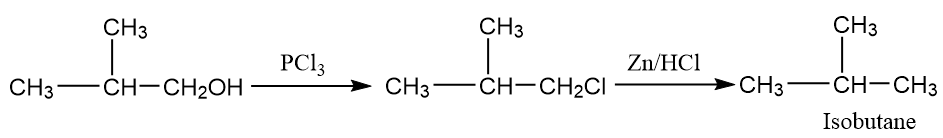Chemical Properties of Alcohols
Question

# ${C}_{4}{H}_{10}O\left(A\right)\text{\hspace{0.17em}}\stackrel{KMn{O}_{4}\text{\hspace{0.17em}}/\text{\hspace{0.17em}\hspace{0.17em}}{H}^{+}}{\to }\text{\hspace{0.17em}\hspace{0.17em}}{C}_{4}{H}_{8}{O}_{2}.$

Moderate
Solution

##Get Instant Solutions